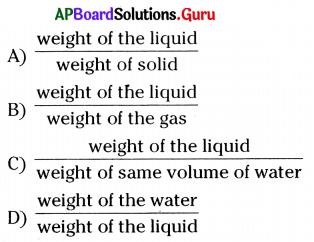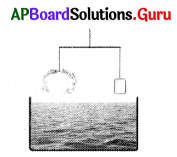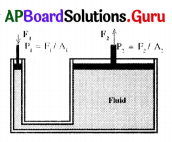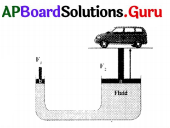Practice the AP 9th Class Physical Science Bits with Answers Chapter 9 Floating Bodies on a regular basis so that you can attempt exams with utmost confidence.

## AP State Syllabus 9th Class Physical Science Bits 9th Lesson Floating Bodies with Answers

Question 1.
Density is defined as
A) mass/litre
B) mass/volume
C) mass/area
D) mass/cross section
B) mass/volume

Question 2.
Unit of density
A) kg/cm3
B) g/m3
C) kg/m3
D) m3/kg
C) kg/m3Question 3.
An object floats on the surface of a liquid when
A) the density of the object is more than the density of liquid
B) the density of the object is less than the density of the liquid
C) weight of the object is more than the weight of the liquid
D) weight of the object is less than the weight of the liquid
B) the density of the object is less than the density of the liquid

Question 4.
Relative density of an object =
A) Density of the object/density of water
B) Density of water/density of the object
C) Weight of the object/weight of the water
D) Volume of the object/volume of the water
A) Density of the object/density of water

Question 5.
Lactometer works on the principle of
A) density
B) relative density
C) buoyancy
D) volume
B) relative density

Question 6.
The objects with relative density greater than 1 ………………… water.
A) floats on
B) sinks in
C) suspends in
D) can’t say
D) can’t say

Question 7.
At normal atmospheric pressure the height of the mercury column in barometer is
A) 76 cm
B) 7.6 cm
C) 76 mm
D) 100 cm
A) 76 cm

Question 8.
The value of one atmospheric pressure is
A) 1.01 × 103 N/m2
B) 1.01 × 104 N/m2
C) 1.01 × 106N/m2
D) 1.01 × 105 N/m2
D) 1.01 × 105 N/m2

Question 9.
Unit of atmospheric pressure is
A) Pascal
B) N/m2
C) A or B
D) None
C) A or BQuestion 10.
Any object immersed in a water experiences an upward force called
A) gravitation
B) buoyancy
C) pressure
D) density
B) buoyancy

Question 11.
Hydraulic jack works on the principle of
A) Archimedes’ principle
B) Buoyancy
C) Pascal’s principle
D) Air pressure
C) Pascal’s principle

Question 12.
Mass per unit volume is known as ……………..
A) density
B) relative density
C) weight
D) pressure
A) density

Question 13.
Unit of density in MKS system = ……………….
A) g/cm3
B) kg/m3
C) g/mm3
D) kg/km3
B) kg/m3

Question 14.
Relative density of an object = …………………
A) $$\frac{\text { density of water }}{\text { density of object }}$$
B) $$\frac{\text { density of object }}{\text { density of water }}$$
C) density of object × density of water
D) none of these
B) $$\frac{\text { density of object }}{\text { density of water }}$$

Question 15.
Relative density of a liquid = ……………………C) $$\frac{\text { weight of the liquid }}{\text { weight of same volume of water }}$$

Question 16.
Units of relative density …………………
A) g/cm3
B) kg/m3
C) kg/cm3
D) no units
D) no unitsQuestion 17.
Lactometer is used to determine the ……………..
A) purity of water
B) purity of milk
C) purity of sugar
D) purity of oil
B) purity of milk

Question 18.
The principle involved in working of a lactometer is …………………
A) density
B) volume
C) relative density
D) pressure
C) relative density

Question 19.
The effective density of a mixture of two liquids of densities ρ1 and ρ2 when they are taken with same masses …………………
A) $$\frac{1}{2}$$ (ρ1 + ρ2)
B) $$\frac{2 \rho_{1} \rho_{2}}{\rho_{1}+\rho_{2}}$$
C) $$\frac{2 \rho_{1} \rho_{2}}{\rho_{1}-\rho_{2}}$$
D) $$\frac{1}{2}$$ (ρ1 – ρ2)
B) $$\frac{2 \rho_{1} \rho_{2}}{\rho_{1}+\rho_{2}}$$

Question 20.
The effective density of a mixture of two liquids of densities ρ1 and ρ2, when they are taken with same volumes is ………………
A) $$\frac{1}{2}$$ (ρ1 + ρ2)
B) $$\frac{2 \rho_{1} \rho_{2}}{\rho_{1}-\rho_{2}}$$
C) $$\frac{1}{2}$$ (ρ1 – ρ2)
D) B) $$\frac{2 \rho_{1} \rho_{2}}{\rho_{1}+\rho_{2}}$$
A) $$\frac{1}{2}$$ (ρ1 + ρ2)

Question 21.
Density of any liquid can be determined by using …………………..
A) hydrometer
B) densitometer
C) hygrometer
D) Both A & B
D) Both A & B

Question 22.
The relative density of an object is greater than 1, then the object …………………. in water.
A) floats
B) sinks
C) both A and B
D) none of these
B) sinks

Question 23.
The upward pressure acting on a body immersed in water is known as ……………….
A) tension
B) normal force
C) buoyancy
D) thurst
C) buoyancyQuestion 24.
1 atmosphere = ………………. N/m2.
A) 1.01 × 105
B) 1.01 × 103
C) 1.01 × 107
D) 1.01 × 107
A) 1.01 × 105

Question 25.
Density of mercury = ………………….
A) 13.6 g/cc
B) 13.6 g/m3
C) 13.6 g/mm3
D) 13.6 kg/m3
B) 13.6 g/m3

Question 26.
Pressure at a depth ‘h’ in a liquid is ………………
A) P = Po + hρg
B) P = Po – hρg
C) Po = P + hρg
D) Po = P – hρg
A) P = Po + hρg

Question 27.
In a bramah press, force acting on the right piston is …………………
A) F2 = $$\frac{\mathrm{A}_{1} \times \mathrm{F}_{1}}{\mathrm{~A}_{2}}$$
B) F2 = $$\frac{\mathrm{F}_{1} \times \mathrm{A}_{2}}{\mathrm{~A}_{1}}$$
C) F2 = $$\frac{A_{2}}{F_{1} \times A_{1}}$$
D) F2 = $$\frac{\mathrm{A}_{1}}{\mathrm{~F}_{1} \times \mathrm{A}_{2}}$$
B) F2 = $$\frac{\mathrm{F}_{1} \times \mathrm{A}_{2}}{\mathrm{~A}_{1}}$$

Question 28.
When a body is immersed in a fluid, the force of buoyancy is equal to the ……………
A) volume of fluid displaced
B) pressure of fluid displaced
C) density of fluid displaced
D) weight of fluid displaced
D) weight of fluid displaced

Question 29.
Relative density of an object is less than 1 then it ………………..
A) floats in water
B) sinks in water
C) both
D) none of these
B) sinks in water

Question 30.
Height of mercury column is ………………..
A) 76 m
B) 76 cm
C) 76 mm
D) Both B & C
D) Both B & CQuestion 31.
Atmospheric pressure Po =
A) hρg
B) $$\frac{\mathrm{h} \rho}{\mathrm{g}}$$
C) $$\frac{\mathrm{hg}}{\mathrm{P}}$$
D) $$\frac{\rho g}{h}$$
A) hρg

Question 32.
Which of the following sinks in water ?
A) wooden block
B) piece of wax
C) glass marble
D) plastic ball
C) glass marble

Question 33.
If we weigh a piece of iron and a piece of wood of same size, iron will weigh more. The reason is
A) density of iron is less than the density of wood
B) weight of iron is more than the weight of wood
C) area of iron is more than the area of wood
D) density of iron is more than the density of wood
D) density of iron is more than the density of wood

Question 34.
Force of buoyancy is equal to the ……………….. of the object.
A) apparent loss of volume
B) apparent loss of density
C) apparent loss of pressure
D) apparent loss of weight
D) apparent loss of weight

Question 35.
Atmospheric pressure can be measured by using
A) lactometer
B) hydrometer
C) barometer
D) hygrometer
C) barometer

Question 36.
Adjacent experiment was done by ancient Greek Scientist.A) Pascal
B) Archimedes
C) Both of them
D) None
B) Archimedes

Question 37.
Name of the arrangement given in the figure.A) Pascal
B) Archimedes
C) Bramah press
D) Hydralic jack
C) Bramah pressQuestion 38.
Name of the set up given in the figure.A) Pascal
B) Archimedes
C) Bramah press
D) Hydralic jack
D) Hydralic jack

Question 39.
Kerosene ………………. water.
A) floats on
B) sinks in
C) suspends in
D) can’t say
A) floats on

Question 40.
Ships are made based on the principle of ………………….
A) pressure
B) volume
C) density
D) buoyancy
D) buoyancy

Question 41.
Hydraulic jacks works on …………………. principle.
A) Archimedes
B) Pascal
C) Berzelius
D) Boyle
B) Pascal

Question 42.
One pascal equal to
A) 1.01 x 105 Nm-2
B) 1.01 x 10-5 Nm-2
C) 1 Nm-2
D) 76 Nm-2
C) 1 Nm-2

Question 43.
The instrument used to test purity of milk is
A) Barometer
B) Hydrometer
C) Potentiometer
D) Lactometer
D) Lactometer

Question 44.
A solid sphere has a radius of 2 cm and a mass of 0.05 kg. What is the relative density of the sphere………………….?
A) 1.39
B) 1.39 kg/m3
C) 1.49
D) 1.46 kg/m3
C) 1.49

Question 45.
Which of the following law denotes Buoyancy ?
A) Pascal’s Law
B) Archimedes Law
C) Boyl’s Law
D) Newton’s Law
B) Archimedes LawQuestion 46.
What happens if water is mixed with milk?
A) The density of the mixture is less than the density of milk
B) The density of the mixture is more than the density of milk
C) The volume of the mixture is more than the volume of milk
D) The volume of the mixture is less than the volume of milk
B) The density of the mixture is more than the density of milk

Question 47.
Density : $$\frac{\mathbf{k g}}{\mathbf{m}^{3}}$$ : :relative density : ………………
A) $$\frac{\mathrm{gm}}{\mathrm{cm}^{3}}$$
B) $$\frac{\mathrm{N}}{\mathrm{m}^{2}}$$
C) $$\frac{\text { pascal }}{\mathrm{m}^{3}}$$
D) No units
D) No units

Question 48.
The instrument which is used to test whether water is added to milk or not is
A) Barometer
B) Lactometer
C) Hydrometer
D) Thermometer